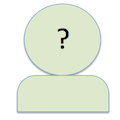#- Page Summarizer Page Summarizer RSS Yioop Software Help Yioop Software Help RSS Yioop Software Help Wiki All Feedsantandt

## Page Summarizer (+0/0). - 22/02/2019Yioop Software Help

I've noticed that there is a "centroid weighted" and a "graph based" summarizer on the page options. I did not see either of these in the docs. What are these two?
Thanks, Anthony
I've noticed that there is a "centroid weighted" and a "graph based" summarizer on the page options. I did not see either of these in the docs. What are these two? Thanks, Anthonyroot

## -- Page Summarizer (+0/0). - 24/02/2019Yioop Software Help

Centroid based summarizers compute an average sentence for a document then add sentences to the summary based on closeness to the average sentence. The basic centroid summarizers doesn't take into account anything about term frequencies within the sentences when computing the average sentence or similarities between sentences and the average sentence, centroid weighted summarizer does, but is slower. Graph based imagines sentences are vertices and that there is an edge between sentences of a given weight based on shared terms. It then does a page rank style computation of the most important sentences. This is the slowest summarizer. In testing, it didn't do much better than centroid weighted summarizer.
Centroid based summarizers compute an average sentence for a document then add sentences to the summary based on closeness to the average sentence. The basic centroid summarizers doesn't take into account anything about term frequencies within the sentences when computing the average sentence or similarities between sentences and the average sentence, centroid weighted summarizer does, but is slower. Graph based imagines sentences are vertices and that there is an edge between sentences of a given weight based on shared terms. It then does a page rank style computation of the most important sentences. This is the slowest summarizer. In testing, it didn't do much better than centroid weighted summarizer.

[X ]